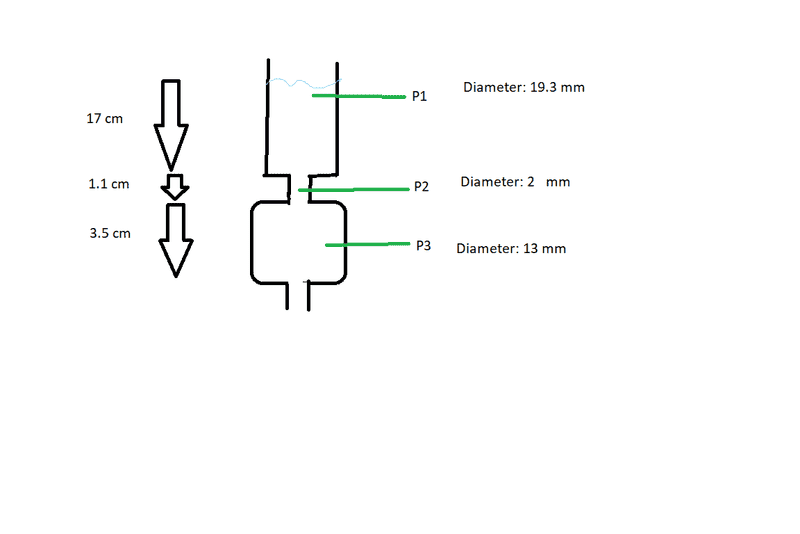# Issue calculating water pressure due to the constriction of water flow

RawPotatoes
For an experiment where I must be able to control the pressure i attached to a syringe a 'chamber'. The inner diameter of the syringe is 19.3 mm and i will fill water to 17 cm of the syringe bottom (i call this P1), the spout has a inner diameter of 2 mm and a length of 2 mm (i call this P2) and the chamber where i want to know the pressure of has an inner diameter of 13 mm and a length of 3.5 cm (i call this P3) but i am interested of the prssure at 1.75 cm.

Water will constantly be flowing trough this setup which will be vertical, i will use a pump to keep the water level at 17 cm from the bottom of the syringe.

Must i take the fact that the diameter of P3 is smaller into consideration or also the fact that the diameter of P3 is smaller than the one of P1? If i ignore all of this and simply calculate the length form the top of the water surface which will be simply open to air to the point that i am interested in i get a pressure of 1.66 kPa (density of water x gravity x length). Any help is apriciated.

•Delta2

Mentor

RawPotatoesI hope that this is clear

•Delta2
Mentor
Is the very bottom open to the air? What is the diameter at the very bottom?

•RawPotatoes
RawPotatoes
Is the very bottom open to the air? What is the diameter at the very bottom?
The bottom is open to air and has the same diameter and length as P2

Mentor
The bottom is open to air and has the same diameter and length as P2
Then, if we apply the Bernoulli equation between the bottom and point p2, we will find a slight negative gauge pressure at point p2 of ##-\rho g h##, where h = 13 mm.

•vanhees71 and RawPotatoes
RawPotatoes
Then, if we apply the Bernoulli equation between the bottom and point p2, we will find a slight negative gauge pressure at point p2 of ##-\rho g h##, where h = 13 mm.

Sorry but i don't understand. The diameter is 13 mm but length is 11 mm. I am trying to calculate the pressure in the middle of P3.

Mentor
Sorry. h=4.05 cm. And you said P2, not P3, right?

•vanhees71 and RawPotatoes
RawPotatoes
Sorry. h=3.5 cm. And you said P2, not P3, right?
I am interested in the pressure at the middle of P3, the total height of P3 is 3.5 cm. What i am asking is if i can simply calculate the pressure from the surface of the water which will be constant. I ask this because i believe that since P2 has a smaller diameter that this will lower the pressure that i need to use a different formula to calculate the pressure in the middle of P3.

Mentor
I am interested in the pressure at the middle of P3, the total height of P3 is 3.5 cm. What i am asking is if i can simply calculate the pressure from the surface of the water which will be constant. I ask this because i believe that since P2 has a smaller diameter that this will lower the pressure that i need to use a different formula to calculate the pressure in the middle of P3.
No. The downward velocity at point P3 wouldn't be significant, so using Bernoulli, P3 is h=18.1+1.75 mm below the upper surface. So, for this point, using Bernoulli, you would treat it as if it is hydrostatic (even though the pressure at P2 is reduced by the higher velocity, this pressure reduction is recovered below P2).